CBSE Class 7CBSE
Share

NCERT solutions for Class 7 Science chapter 13 - Motion and Time

Science Textbook for Class 7

NCERT Science Class 7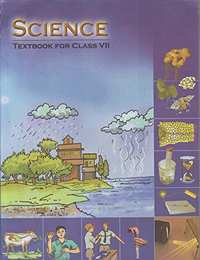Chapter 13: Motion and Time

Chapter 13: Motion and Time solutions [Pages 156 - 1567]

Q 1.1 | Page 156

Classify the given motion along a straight line, circular or oscillatory motion:

Motion of your hands while running.

Q 1.2 | Page 156

Classify the given motion along a straight line, circular or oscillatory motion:

Motion of a horse pulling a cart on a straight road.

Q 1.3 | Page 156

Classify the given motion along a straight line, circular or oscillatory motion:

Motion of a child in a merry-go-round.

Q 1.4 | Page 156

Classify the given motion along a straight line, circular or oscillatory motion:

Motion of a child on a see-saw.

Q 1.5 | Page 156

Classify the given motion along a straight line, circular or oscillatory motion:

Motion of the hammer of an electric bell.

Q 1.6 | Page 156

Classify the given motion along a straight line, circular or oscillatory motion:

Motion of a train on a straight bridge.

Q 2 | Page 156

Which of the following are not correct?

(1) The basic unit of time is second.

(2) Every object moves with a constant speed.

(3) Distances between two cities are measured in kilometres.

(4) The time period of a given pendulum is not constant.

(5) The speed of a train is expressed in m/h.

Q 3 | Page 156

A simple pendulum takes 32 s to complete 20 oscillations. What is the time period of the pendulum?

Q 4 | Page 156

The distance between two stations is 240 km. A train takes 4 hours to cover this distance. Calculate the speed of the train.

Q 5 | Page 156

The odometer of a car reads 57321.0 km when the clock shows the time 08:30 AM. What is the distance moved by the car, if at 08:50 AM, the odometer reading has changed to 57336.0 km? Calculate the speed of the car in km/min during this time. Express the speed in km/h also.

Q 6 | Page 156

Salma takes 15 minutes from her house to reach her school on a bicycle. If the bicycle has a speed of 2 m/s, calculate the distance between her house and the school.

Q 7.1 | Page 156

Show the shape of the distance-time graph for the motion in the following cases:

A car moving with a constant speed.

Q 7.1 | Page 1567

Show the shape of the distance-time graph for the motion in the following cases:

A car parked on a side road.

Q 8 | Page 156

Which of the following relations is correct?

• Speed = Distance × Time

• 2) Speed = "Distance"/"Time"

• Speed = "Time"/"Distance"

• Speed = 1/"Distnace x Time"

Q 9 | Page 157

The basic unit of speed is:

• km/min

• m/min

• km/h

• m/s

Q 10 | Page 157

A car moves with a speed of 40 km/h for 15 minutes and then with a speed of 60 km/h for the next 15 minutes. The total distance covered by the car is:

• 100 km

• 25 km

• 15 km

• 10 km

Q 11 | Page 157

Suppose the two photographs, shown in Figure 1 and Figure 2, had been taken at an interval of 10 seconds. If a distance of 100 metres is shown by 1 cm in these photographs, calculate the speed of the blue car.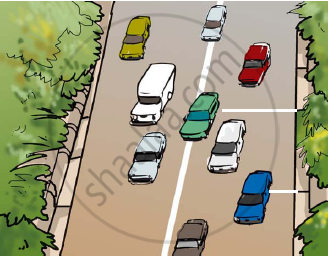Figure 1 Vehicles moving in the same direction of on a road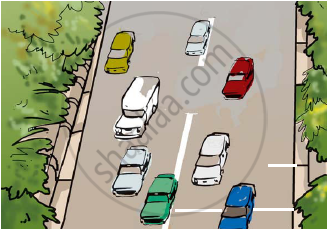Figure 2 Position of vehicles shown in Figure 1 after some time

Q 12 | Page 157

Figure shows the distance-time graph for the motion of two vehicles A and B. Which one of them is moving faster?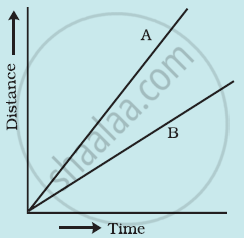Figure: Distance-time graph for the motion of two cars

Q 13 | Page 157

Which of the following distance-time graphs shows a truck moving with speed which is not constant?

•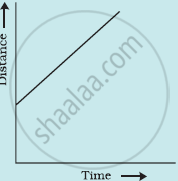•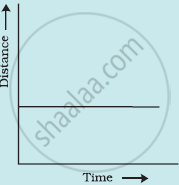•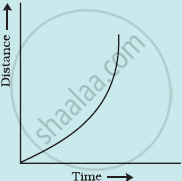•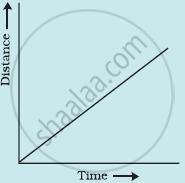Chapter 13: Motion and Time

NCERT Science Class 7NCERT solutions for Class 7 Science chapter 13 - Motion and Time

NCERT solutions for Class 7 Science chapter 13 (Motion and Time) include all questions with solution and detail explanation. This will clear students doubts about any question and improve application skills while preparing for board exams. The detailed, step-by-step solutions will help you understand the concepts better and clear your confusions, if any. Shaalaa.com has the CBSE Science Textbook for Class 7 solutions in a manner that help students grasp basic concepts better and faster.

Further, we at Shaalaa.com are providing such solutions so that students can prepare for written exams. NCERT textbook solutions can be a core help for self-study and acts as a perfect self-help guidance for students.

Concepts covered in Class 7 Science chapter 13 Motion and Time are Group of Motion: Slow Or Fast, Concept of Speed, Measurement of Time, Concept of Measuring Speed, Distance-Time Graph.

Using NCERT Class 7 solutions Motion and Time exercise by students are an easy way to prepare for the exams, as they involve solutions arranged chapter-wise also page wise. The questions involved in NCERT Solutions are important questions that can be asked in the final exam. Maximum students of CBSE Class 7 prefer NCERT Textbook Solutions to score more in exam.

Get the free view of chapter 13 Motion and Time Class 7 extra questions for Science and can use Shaalaa.com to keep it handy for your exam preparation

S